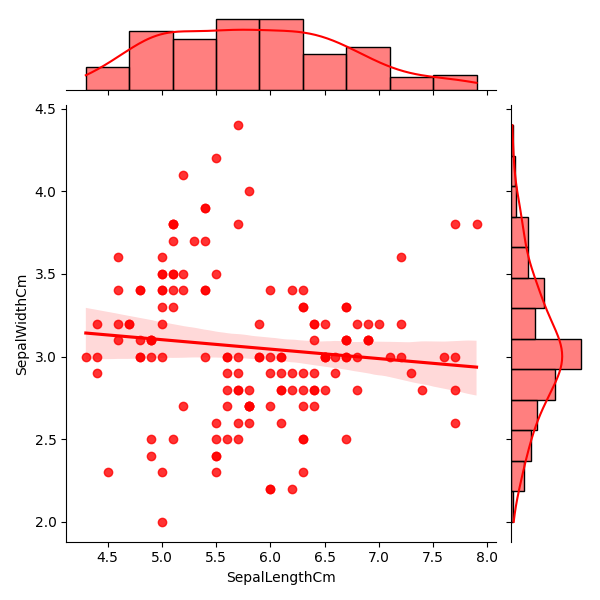﻿ Python Machine learning Scikit-learn: Create a joinplot and add regression and kernel density fits using “reg” to describe individual distributions on the same plot between Sepal length and Sepal width - w3resource# Python Scikit-learn: Create a joinplot and add regression and kernel density fits using “reg” to describe individual distributions on the same plot between Sepal length and Sepal width

## Python Machine learning Iris Visualization: Exercise-10 with Solution

Write a Python program to create a joinplot and add regression and kernel density fits using “reg” to describe individual distributions on the same plot between Sepal length and Sepal width.

Sample Solution:

Python Code:

``````import pandas as pd
import seaborn as sns
import matplotlib.pyplot as plt
fig=sns.jointplot(x='SepalLengthCm', y='SepalWidthCm', kind="reg", color='red', data=iris)
plt.show()
```
```

Sample Output:

``````

Python Code Editor:

Have another way to solve this solution? Contribute your code (and comments) through Disqus.

What is the difficulty level of this exercise?

﻿